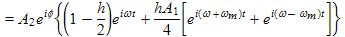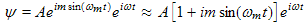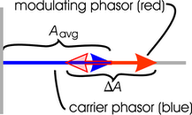There are all sorts of resonances around us, in the world, in our culture, and in our technology. A tidal resonance causes the 55 foot tides in the Bay of Fundy. Mechanical and acoustical resonances and their control are at the center of practically every musical instrument that ever existed. Even our voices and speech are based on controlling the resonances in our throat and mouth. Technology is also a heavy user of resonance. All clocks, radios, televisions, and gps navigating systems use electronic resonators at their very core. Doctors use magnetic resonance imaging or MRI to sense the resonances in atomic nuclei to map the insides of their patients. In spite of the great diversity of resonators, they all share many common properties. In this blog, we will delve into their various aspects. It is hoped that this will serve both the students and professionals who would like to understand more about resonators. I hope all will enjoy the animations.

Origins of Newton's laws of motion

History of mechanical clocks with animations
Understanding a mechanical clock with animations
includes pendulum, balance wheel, and quartz clocks

## Friday, April 1, 2011

### 2.9 Modulation, application of phasor multiplication

This posting includes flash animations showing the physics discussed. Most computers have a flash player already installed, but if yours does not, download the free Adobe flash player here.
 Figure 1. Amplitude modulation (AM modulation) as applied to a trumpet. Mouse over the animation to start it or click on it to restart it. Figure 2. Electronic AM modulation of a high frequency radio signal (the carrier) using a signal from a microphone as the modulating waveform. Mouse over the animation to set it in motion. The high frequency carrier, when launched by the proper antenna, will propagate and is readily detectable over long distances through the atmosphere, whereas the raw microphone signal is not. Click on "show envelope" to see the envelope of the final signal. Note that this envelope has the same shape as the signal from the microphone. The envelope is not part of the real waveform, but is shown to allow you to more easily see the amplitude as a function of time in the animation. In a real radio station, the carrier signal is 100 or more times higher in frequency than the modulation frequency (instead of the factor of eight shown here). Also note that the sinusoids, as shown, are graphical representations of the invisible electronic signals in the wires leading into and out of the mixer. These would require an oscilloscope to make them visible.

## 2.9 Modulation, application of phasor multiplication

Modulation is a key component in electronic communication. Our radios, televisions, cell phones, optical fibers, and satellite links all rely on modulation to transfer information to electromagnetic waves that propagate from one point to another. The math involved with modulation involves multiplying phasors and is a great application of the concepts of phasor multiplication discussed in the last section. In this section we will cover the two simplest forms of modulation: AM and FM modulation.

### AM Modulation

The simplest and earliest form of modulation is amplitude modulation (better known as AM modulation). It simply involves manipulating the amplitude, i.e. envelope, of a sinusoidal signal using the signal that one wishes to send. For example, in the trumpet animation above (Fig. 1), the musician uses his hand to modulate the sound wave in a sinusoidal fashion.

In Fig. 2, we see a complicated voice signal from a microphone modulating a sinusoid and making the envelope of the resulting sinusoid take on the shape of the microphone signal. The resulting modulated wave is suitable for radio transmission. This is the modulation method used for AM radios. The "mixer" shown in this illustration, electronically multiplies the carrier signal with the microphone signal to produce the resulting modulated waveform. The multiplication may be partial (some fraction of the carrier signal gets through without multiplication) or total, depending on the modulation index, h. See Wikipedia and Microwave101 for more on (multiplicative) mixers. In Table 1 below, we show the math for this multiplication.

 Table 1. Math for AM modulation, an example of phasor multiplication. See the previous section for details of this method. To keep the math simple, we consider a carrier signal modulated by a single lower frequency modulation signal (which might be from a microphone as shown in Fig. 2 above). The modulating signal in both real and complex forms is: The carrier signal in both real and complex forms is: The modulated signal in complex form is: where I have shown the troublesome phasor product using the × symbol. h is the fraction of the carrier signal modulated. Substituting (1a) and (1b)into (1c) yields:(1d) again using the × symbol. Next I use the method from the previous section. As discussed there, I need to make one of the two phasors in the product real so as to get a valid result. I choose to make the less complicated modulation signal real (only in the second term that has a phasor multiplication):(1e) I expand (1f) to get:(1g)(1h) Note that the last equation clearly shows that there are three frequencies associated with the modulated signal: the carrier (the first term), the sum frequency (the second term) and the difference frequency (the third term). The carrier (first term) disappears if h = 200% = 2.0 . See homestead.com for an adjustable AM modulation applet.
 Figure 3. Frequency modulation (FM modulation) as applied to a singer. Mouse over the animation to start it or click on it to restart it. If you look closely you can see that the wave crests are more bunched together as the snake squeezes and the singer's voice goes to a higher pitch. Note that the amplitude of the waves is constant.Figure 4. Electronic circuit for frequency modulation (FM modulation). The key component is the voltage controlled oscillator, whose output frequency is controlled by the voltage of the input signal.

### FM Modulation

FM modulation involves modulating the frequency or tone of a constant amplitude signal, and not its amplitude, as seen in the animation to the right. The modulation is harder to see in the signals than AM modulation.

The subject of FM modulation requires understanding of the distinction between instantaneous frequency and Fourier frequency.

Instantaneous frequency is the rate at which a signal is going through phase. That is, if we express a sinusoid as:, then the instantaneous frequency iswhich can vary with time, and does vary in the case of FM modulation.

Fourier frequency relates to taking the spectrum of a complex signal such as a modulated signal. Each Fourier frequency component is a constant sinusoid, constant in magnitude and in frequency. Fourier analysis is a mathematical process for breaking up a complex signal into a sum of these constant Fourier components. Electronic devices, such as radio, television, and cell phone receivers are designed to select particular Fourier components (i.e. frequency bands) out of the jungle of electromagnetic radiation that exists around us.

In Table 2 (next), we show the math for FM modulation.

 Table 2. Math for FM modulation. If we modulate the frequency as shown above, we are modulating the rate of phase increase, not the total accumulated phase. That is to say, instead of an equation like: we need an integral to figure out the accumulated phase, time interval by time interval: where the function "exp(x)" is just another way of writing e raised to the power x. Equation (2a) is similar to that required to calculate the position of an automobile as a function of time starting with the equation for its speed as a function of time. Integrating (2a) yields:.   (2b) Equation (2b) shows that FM modulation is essentially the same as phase modulation, that a modulation of the instantaneous frequency produces a modulation of the phase of a signal. That is to say, (2b) shows a time oscillating term added to the "ωt" in the exponent of e. Assuming that the modulation is small, i.e. that m << 1, then we can use the first two terms of a Taylor's series as follows: Equation (2c) comes from (2a) in which we used a complex phasor along with a real sinusoidally varying phase. This is not the case of a multiplication of two complex phasors, so we can use it "as is" to express the final resulting complex phasor. Equation (2c) is similar to Equation (1f) above for AM modulation, except that there is an "i" before the modulation term, indicating a 90° phase sift between the carrier and modulation. This means that the modulation does not effectively add to the amplitude but instead modulates the phase. We explain this more in the middle of Table 3 below. AM modulation adds a modulation signal in phase with the carrier, whereas FM modulation adds a modulation signal 90° out of phase with the signal. Because a constant frequency noise signal can beat with a carrier and produce AM modulation, FM modulation is a little less affected by noise (at least of this type). Quadrature modulation combines both AM and FM modulation for maximum information transfer for a given bandwidth. To find the Fourier frequencies of FM modulation we convert the sin in (2c) into its complex form (this is not a conversion to a complex phasor, but instead simply an exact substitution of one form of a sin function into another form of it): We see the two frequencies of the sidebands similar to the AM modulation case above, i.e. there is a sum of frequencies term and a difference of frequencies term. These are graphed at the bottom of Table 3 below. If we had done the math for larger modulation amplitudes (larger m)and not used the approximation in (2c), then we would have gotten more sidebands and the math would have involved Bessel functions as summarized in Wikipedia. Go to homestead.com for an adjustable applet showing FM modulation.

### Comparison of the two modulation methods

Table 3. Comparison of AM and FM modulation
AM modulation FM modulation
Elemental modulation circuitsElemental modulation equations
(from Equations (1f) and (2c) above.)(3a)

As shown in the phasor diagram below, the two terms inside the curly brackets are both real and directly add (or subtract when one is negative). The modulating signal is in phase with the carrier signal). Thus the red modulating signal modulates the amplitude of the carrier.(3b)

One term inside the brackets is real and one is imaginary, meaning that one is shifted in phase by 90° from the other. As shown in the phasor diagram below, this leads to the modulating signal varying the phase of the carrier.
Phasor diagramsWe do a phasor diagram for everything inside the curly brackets in Equation (3a) which shows how the vectors add relative to each other with the common factors taken out. In this diagram, the carrier term is constant and is shown as the constant blue phasor. Relative to this constant carrier vector, the phasor for the modulating term is shown in red and has a cosωt dependence that makes it vary sinusoidally in the real direction (horizontally) as shown. It is in phase with the carrier signal. When we add the two phasors, the resulting phasor is constant in direction, horizontally (i.e. in phase) and oscillates in magnitude (i.e. amplitude).Here we draw the phasor addition for everything inside the brackets in Equation (3b) above. The phasor for the carrier term in Equation (3b) is shown in blue and is constant in the real direction or horizontally. The modulating term is imaginary with a sine dependence and so is shown in red as a vertical oscillating phasor (90° out of phase with respect to the carrier signal). When we add the two phasors, the resulting phasor is nearly constant in magnitude (i.e. amplitude) and oscillates in direction (i.e. phase or in θ) as required for FM and phase modulation.

Comparing Equations (3a) and (3b) we see that they are very similar with the main difference being that the modulated term (the second term) of the AM case is in phase with the carrier, while it is 90° out of phase with the carrier in the FM case.

Fourier series equationsComparing the two Fourier series equations in the AM and FM cases, we see that they are almost identical in form. The main difference is that there is a plus sign between the sum and difference frequency terms in the AM case whereas there is a minus sign in the same position in the FM case.

Fourier series strengths(Note: the two graphs here are the same. In general they might have different ratios of carrier to sideband strengths, depending on the modulation strengths in the two cases. There is a difference in the phase relationships between the two sidebands in the two modulation methods as noted in the text for the Fourier series equations, just above.)
Spectrum when modulating signal is composed of a range of frequencies, as is usually the case, such as the spectrum of the microphone signal shown below.Spectrum of the resulting modulated signal. As in the case above, the spectra for both AM and FM modulations are the same (for small FM modulations).Summary

• Amplitude modulation (AM modulation) consists of varying the amplitude of a sinusoidal signal called a "carrier signal". The amplitude at each instant in time is controlled by the strength of a modulating signal at that instance.

• The resulting spectrum consists of the carrier signal and two sidebands. These have frequencies equal to that of the carrier signal minus that of the modulating signal and that of the carrier signal plus that of the modulating signal.

• Our scheme for multiplying two phasors proved useful for calculating the resulting modulated waveform.

• Frequency modulation (FM modulation) consists of varying the instantaneous frequency of an oscillator in response to the instantaneous strength of a modulating signal.

• For small modulations, the resulting spectrum consists of the center frequency plus two sidebands. The sidebands have frequencies equal to the center frequency minus the frequency of the modulating signal and the center frequency plus the modulating frequency.

• Our scheme for multiplying phasors was less central in the case of FM modulation, but still helped us understand what we were doing.

• If the modulating signal contains a range of frequencies as is the usual case, then the side bands would each be broadened to contain the same width of frequencies.

P. Ceperley Feb. 2010